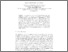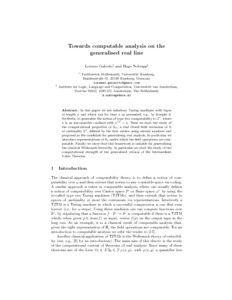# PP-2017-08: Towards computable analysis on the generalised real line

PP-2017-08: Galeotti, Lorenzo and Nobrega, Hugo (2017) Towards computable analysis on the generalised real line. [Pre-print] (In Press)Preview Text Towards_computable_analysis_on_the_generalised_real_line.pdf Download (317kB) | Preview
Official URL: http://www.springer.com/gp/book/9783319587400

## Abstract

In this paper we use infinitary Turing machines with tapes of length $\kappa$ and which run for time $\kappa$ as presented, e.g., by Koepke \& Seyfferth, to generalise the notion of type two computability to $\cantor$, where $\kappa$ is an uncountable cardinal with $\kappa^{<\kappa}=\kappa$. Then we start the study of the computational properties of $\mathbb{R}_\kappa$, a real closed field extension of $\mathbb{R}$ of cardinality $2^\kappa$, defined by the first author using surreal numbers and proposed as the candidate for generalising real analysis. In particular we introduce representations of $\mathbb{R}_\kappa$ under which the field operations are computable. Finally we show that this framework is suitable for generalising the classical Weihrauch hierarchy. In particular we start the study of the computational strength of the generalised version of the Intermediate Value Theorem.

Item Type: Pre-print PP-2017-08 Prepublication (PP) Series 2017 Best student paper award. ComputationLogicMathematics Hugo Nobrega 07 May 2017 08:09 07 May 2017 08:09 https://eprints.illc.uva.nl/id/eprint/1536View Item